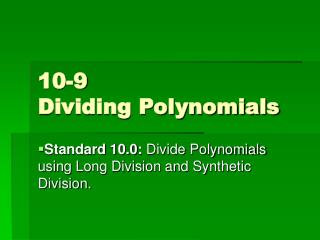DownloadDownload Presentation10-9 Dividing Polynomials

# 10-9 Dividing Polynomials

Télécharger la présentation## 10-9 Dividing Polynomials

- - - - - - - - - - - - - - - - - - - - - - - - - - - E N D - - - - - - - - - - - - - - - - - - - - - - - - - - -
##### Presentation Transcript

1. 10-9Dividing Polynomials Standard 10.0: Divide Polynomials using Long Division and Synthetic Division.

2. #1 Divide a polynomial by a monomial.

3. #2 Divide a polynomial by a monomial.

4. Long Division • Divide the first term of the numerator by the first term of the denominator. • Distribute your quotient by the entire polynomial in the denominator. • Distribute a negative by through your product.

5. #7 Dividing a polynomial by a polynomial (Long Division)

6. #8 Dividing a polynomial by a polynomial (Long Division)